#Quadratic Polynomials: Factoring by GuessingThere are three methods to factor a quadratic polynomial: Factoring by guessing, "completing the square", and the quadratic formula. On this page you will learn the first method. The next two pages are devoted to the other methods.

You may also want to visit the S.O.S. Mathematics section The Quadratic Equation.

#### A first example.

Consider the polynomial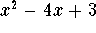. If it is reducible, the answer will look like this: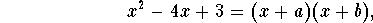the catch being that we do not know the roots -a and -b. One popular trick in Mathematics is to work backwards: Using the FOIL method we can write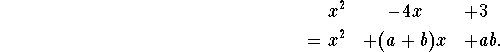From this we see that the two unknowns a and b have to satisfy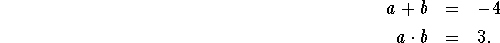In English: we need two numbers whose product is 3 and whose sum is -4. At this point, start guessing using integer values for a and b. There are really only two choices which come to mind: Among integers only the pair 1 and 3 and the pair -1 and -3 have product 3. Checking their sums we see that we have to have a=-1 and b=-3 (or vice versa). We are done: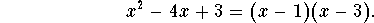#### Another example.

Let's try with the polynomial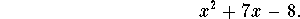We want to find integers a and b whose sum is 7 and whose product is -8. There are four choices for two integers with product -8: a=1, b=-8; a=-1, b=8; a=2, b=-4 and a=-2 and b=4. Among these, only a=-1, b=+8 has the required sum 7. We are done: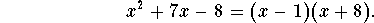#### What is the catch?

This method is amazingly simple; with some experience it will take you no longer than a couple of seconds to come up with a factorization, but only if this method works. In reality, chances are pretty slim that a random polynomial, even with integer coefficients, will have integer roots (and we are restricting our search for a and b to integers!); in the classroom, your chances are better: most teachers are nice (and/or lazy?), so quite a few polynomials you see on the blackboard can probably be factored by guessing.

#### A last example.

Let's try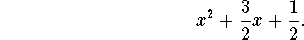Did you already gain some practice? Even though the roots are rational numbers instead of integers, I hope the solution will "jump at you" after staring at the polynomial for a few seconds: a=1 and b=1/2 will work: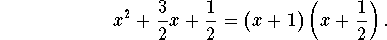#### Exercise 1.

Which of the following polynomial can be factored by the guessing method? Give the factorization in these cases!
•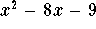•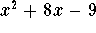•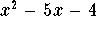•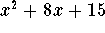•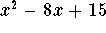[Back] [Next]
[Algebra] [Trigonometry] [Complex Variables]
[Calculus] [Differential Equations] [Matrix Algebra]S.O.S MATHematics home page

Do you need more help? Please post your question on our S.O.S. Mathematics CyberBoard.Helmut Knaust